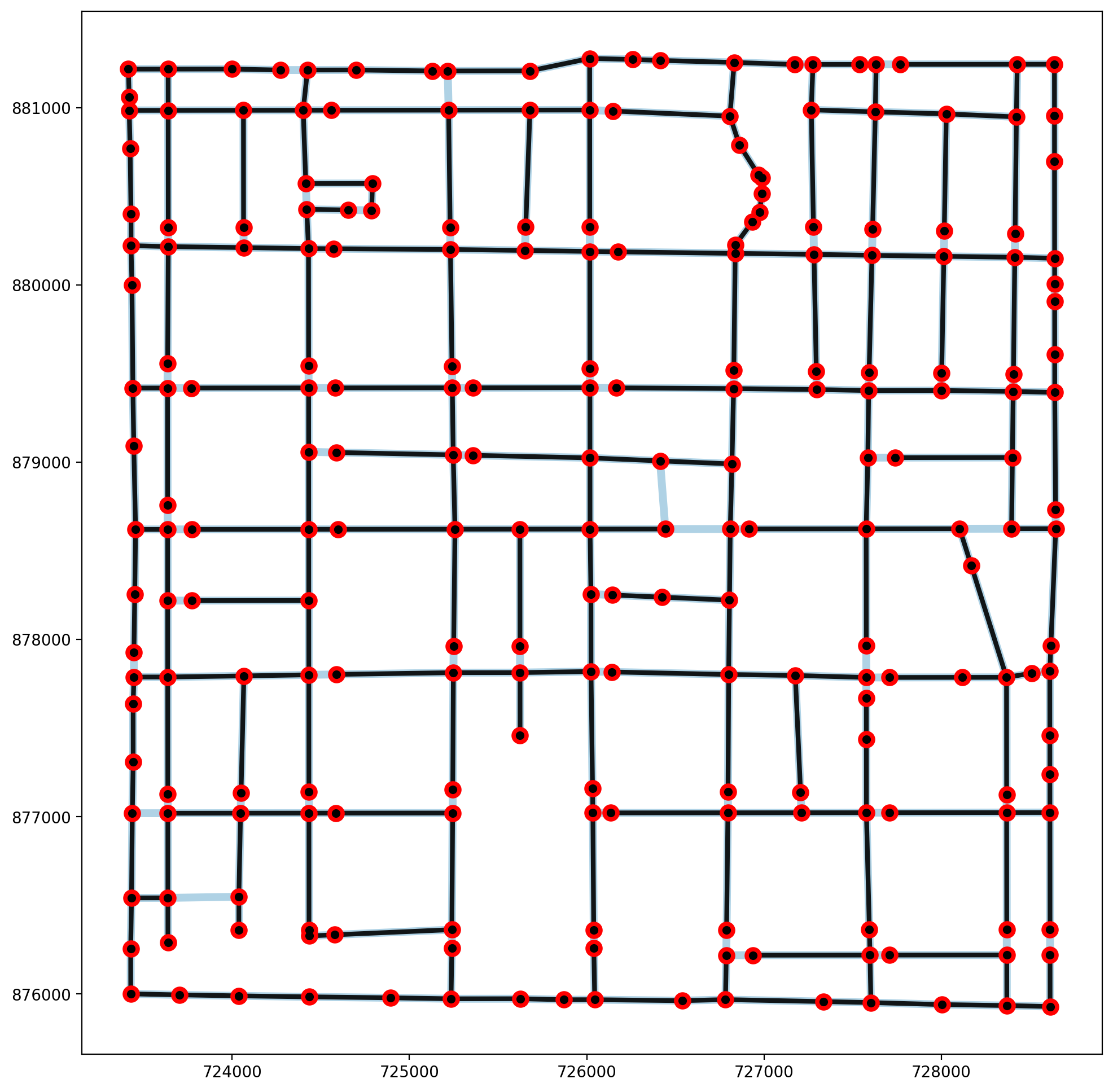If any part of this notebook is used in your research, please cite with the reference found in README.md.

# Network spanning trees¶

## Understanding minimum & maximum spanning trees in spaghetti¶

Author: James D. Gaboardi jgaboardi@gmail.com

This notebook demonstrates minimum & maximum spanning trees for the following:

1. Elementary geometric objects

2. Synthetic networks

3. An empirical example

:

%config InlineBackend.figure_format = "retina"

:

%load_ext autoreload
%watermark

Last updated: 2022-04-15T11:21:52.473551-04:00

Python implementation: CPython
Python version       : 3.10.4
IPython version      : 8.2.0

Compiler    : Clang 12.0.1
OS          : Darwin
Release     : 21.4.0
Machine     : x86_64
Processor   : i386
CPU cores   : 8
Architecture: 64bit


:

import geopandas
import libpysal
from libpysal import cg
import matplotlib
import matplotlib.pyplot as plt
import spaghetti

%matplotlib inline
%watermark -w
%watermark -iv

Watermark: 2.3.0

libpysal  : 4.6.2
geopandas : 0.10.2
json      : 2.0.9
matplotlib: 3.5.1
spaghetti : 1.6.5+44.g17b88a0.dirty


/Users/the-gaboardi/spaghetti/spaghetti/network.py:36: FutureWarning: The next major release of pysal/spaghetti (2.0.0) will drop support for all libpysal.cg geometries. This change is a first step in refactoring spaghetti that is expected to result in dramatically reduced runtimes for network instantiation and operations. Users currently requiring network and point pattern input as libpysal.cg geometries should prepare for this simply by converting to shapely geometries.
warnings.warn(f"{dep_msg}", FutureWarning)


### Helper functions for plotting and labeling¶

:

def plotter(net_arcs, net_verts, mst_arcs=None, mst_verts=None, label=True):
"""Convenience plotting function."""
plot_mst, msize, vert_z = False, 40, 3
if hasattr(mst_arcs, "T") and hasattr(mst_verts, "T"):
plot_mst, msize, vert_z = True, 20, 4
# set arc keyword arguments
arc_kws = {"column":"comp_label", "cmap":"Paired"}
# set the streets as the plot base
base_kws = {"figsize":(12, 12)}
base_kws.update(arc_kws)
base = net_arcs.plot(lw=5, alpha=.9, **base_kws)
# create vertices keyword arguments for matplotlib
ax_kwargs = {"ax":base}
net_verts.plot(color="k", markersize=msize, zorder=vert_z, **ax_kwargs)
# plot spanning trees
if plot_mst:
mst_arcs.plot(color="k", lw=3, zorder=2, alpha=.9, **ax_kwargs)
mst_verts.plot(color="r", markersize=100, zorder=3, **ax_kwargs)
# label network/tree elements
if label:
if not plot_mst:
arc_labels(net_arcs, base, 12)
vert_labels(net_verts, base, 14)
else:
arc_labels(mst_arcs, base, 12)
vert_labels(mst_verts, base, 14)

def arc_labels(a, b, s):
"""Label each network arc."""
def _lab_loc(_x):
"""Helper for labeling network arcs."""
return _x.geometry.interpolate(0.5, normalized=True).coords
kws = {"size": s, "ha": "center", "va": "bottom"}
a.apply(lambda x: b.annotate(text=x.id, xy=_lab_loc(x), **kws), axis=1)

def vert_labels(v, b, s):
"""Label each network vertex."""
def _lab_loc(_x):
"""Helper for labeling vertices."""
return _x.geometry.coords
kws = {"size": s, "ha": "left", "va": "bottom", "weight": "bold"}
v.apply(lambda x: b.annotate(text=x.id, xy=_lab_loc(x), **kws), axis=1)


## 1. Elementary geometric objects¶

### 1.a Simple cross¶

:

bounds = (0,0,2,2)
h, v = 1, 1
cross = spaghetti.regular_lattice(bounds, h, nv=v, exterior=False)
lines = geopandas.GeoDataFrame(geometry=cross)

:

ntw = spaghetti.Network(in_data=lines)
elem_kws = {"vertices":True, "arcs":True}
vertices, arcs = spaghetti.element_as_gdf(ntw, **elem_kws)

:

plotter(arcs, vertices, mst_arcs=None, mst_verts=None)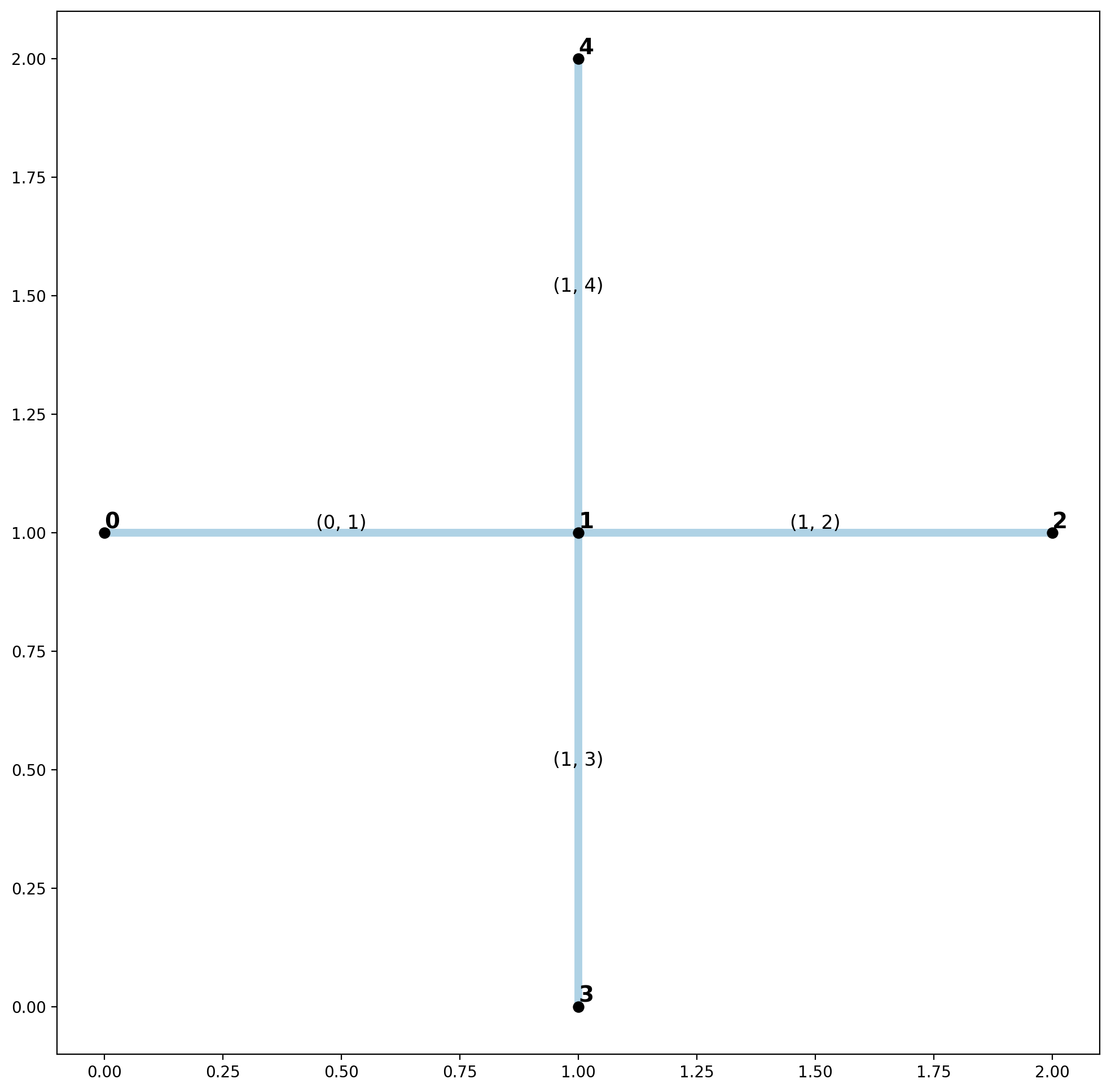Minimum Spanning Tree

:

minst_net = spaghetti.spanning_tree(ntw)
mst_verts, mst_arcs = spaghetti.element_as_gdf(minst_net, **elem_kws)
plotter(arcs, vertices, mst_arcs=mst_arcs, mst_verts=mst_verts)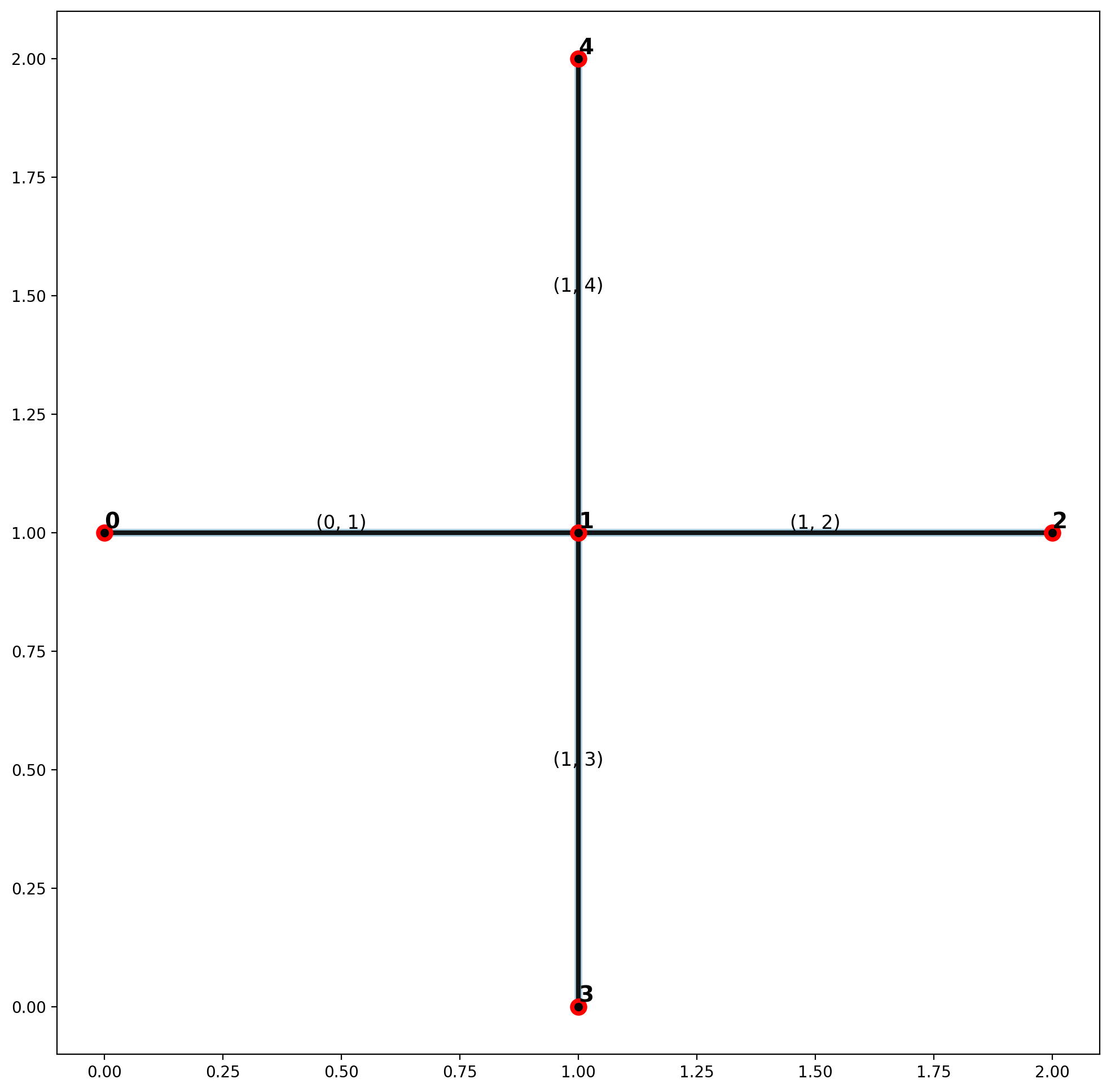Maximum Spanning Tree

:

maxst_net = spaghetti.spanning_tree(ntw, maximum=True)
mst_verts, mst_arcs = spaghetti.element_as_gdf(maxst_net, **elem_kws)
plotter(arcs, vertices, mst_arcs=mst_arcs, mst_verts=mst_verts)No cycles can be formed with this simple intersection. Therefore, all network arcs are both members of the minimum and maximum spanning trees.

### 1.b Pythagorean triple triangle¶

:

p00 = cg.Point((0,0))
lines = [cg.Chain([p00, cg.Point((0,3)), cg.Point((4,0)), p00])]

:

ntw = spaghetti.Network(in_data=lines)
elem_kws = {"vertices":True, "arcs":True}
vertices, arcs = spaghetti.element_as_gdf(ntw, **elem_kws)

:

plotter(arcs, vertices, mst_arcs=None, mst_verts=None)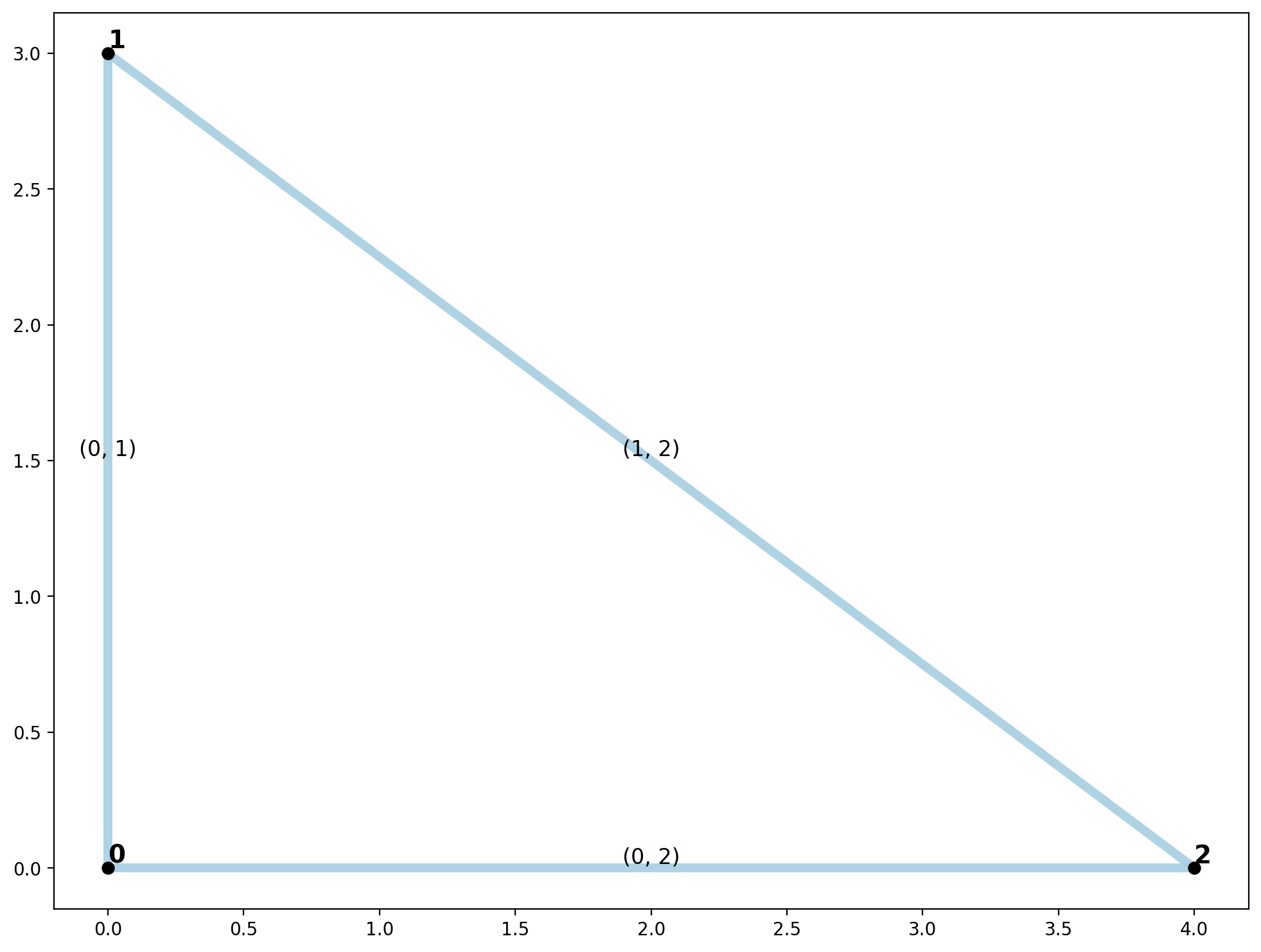Minimum Spanning Tree

:

minst_net = spaghetti.spanning_tree(ntw)
mst_verts, mst_arcs = spaghetti.element_as_gdf(minst_net, **elem_kws)
plotter(arcs, vertices, mst_arcs=mst_arcs, mst_verts=mst_verts)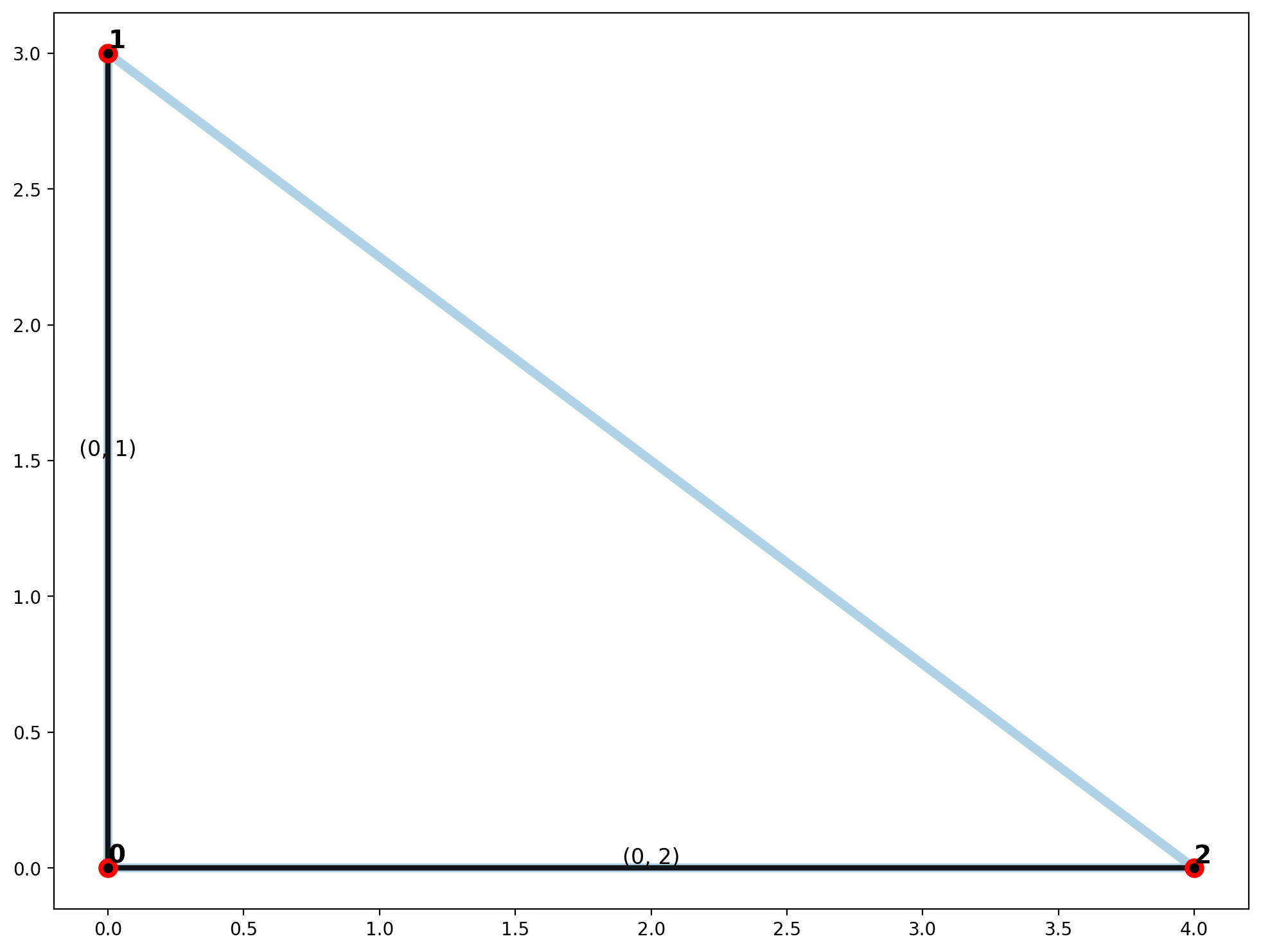Maximum Spanning Tree

:

maxst_net = spaghetti.spanning_tree(ntw, maximum=True)
mst_verts, mst_arcs = spaghetti.element_as_gdf(maxst_net, **elem_kws)
plotter(arcs, vertices, mst_arcs=mst_arcs, mst_verts=mst_verts)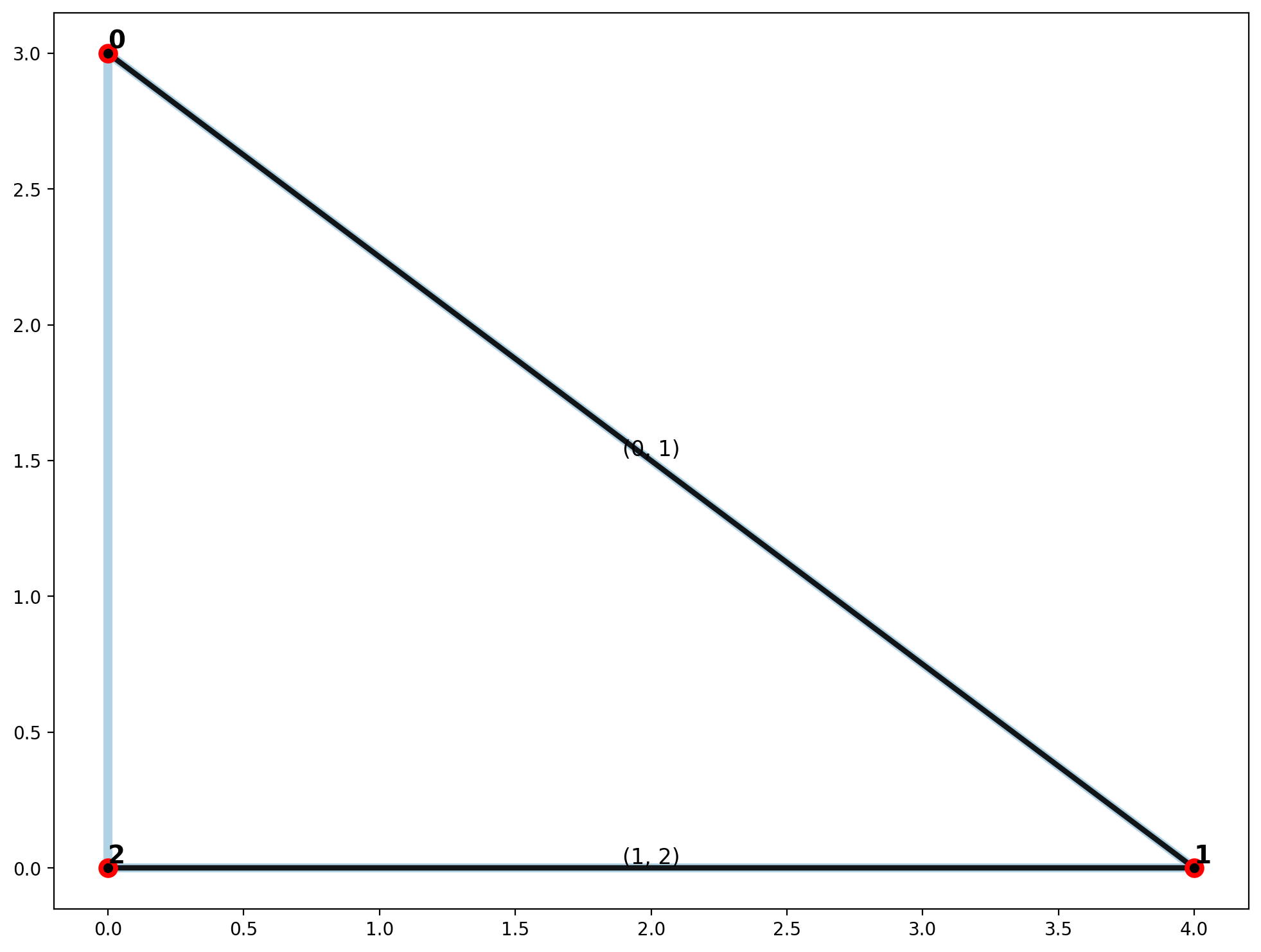Due to the nature of a Pythagorean triple triangle, it is excellent for demonstrating the most basic example of a network cycle, and the difference between a minimum and maximum spanning tree.

## 2. Synthetic Networks¶

### 2.a Inspired by Figure 3.25 in Okabe and Sugihara (2012)¶

:

p04, p94, p90 = cg.Point((0,4)), cg.Point((9,4)), cg.Point((9,0))
p33, p43 = cg.Point((3,3)), cg.Point((4,3))
# interior
lines = [cg.Chain([p04, p33, p00]), cg.Chain([p94, p43, p90]), cg.Chain([p33, p43])]
# exterior
lines += [cg.Chain([p00, p04, p94, p90, p00])]

:

ntw = spaghetti.Network(in_data=lines)
elem_kws = {"vertices":True, "arcs":True}
vertices, arcs = spaghetti.element_as_gdf(ntw, **elem_kws)

:

plotter(arcs, vertices, mst_arcs=None, mst_verts=None)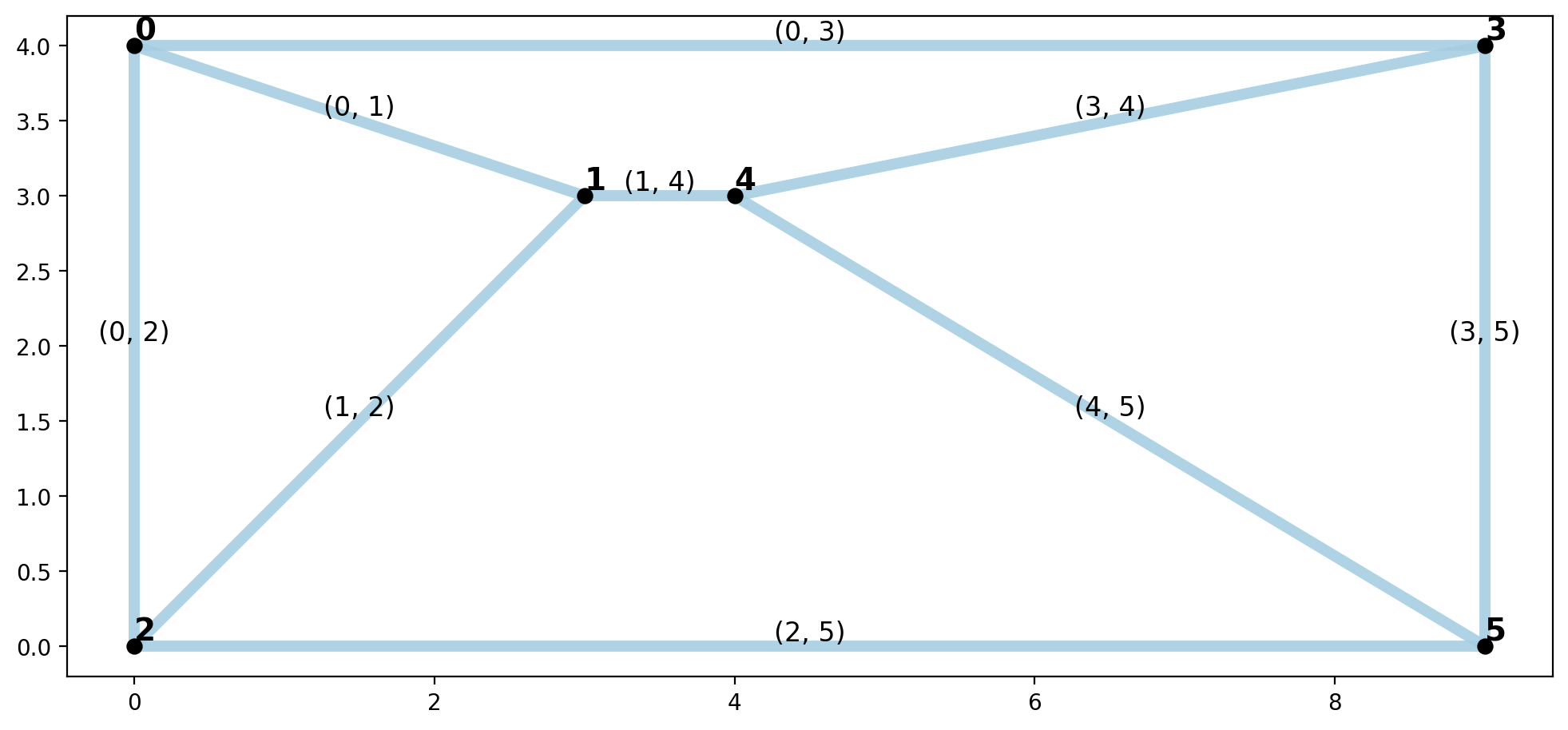Minimum Spanning Tree

:

minst_net = spaghetti.spanning_tree(ntw)
mst_verts, mst_arcs = spaghetti.element_as_gdf(minst_net, **elem_kws)
plotter(arcs, vertices, mst_arcs=mst_arcs, mst_verts=mst_verts)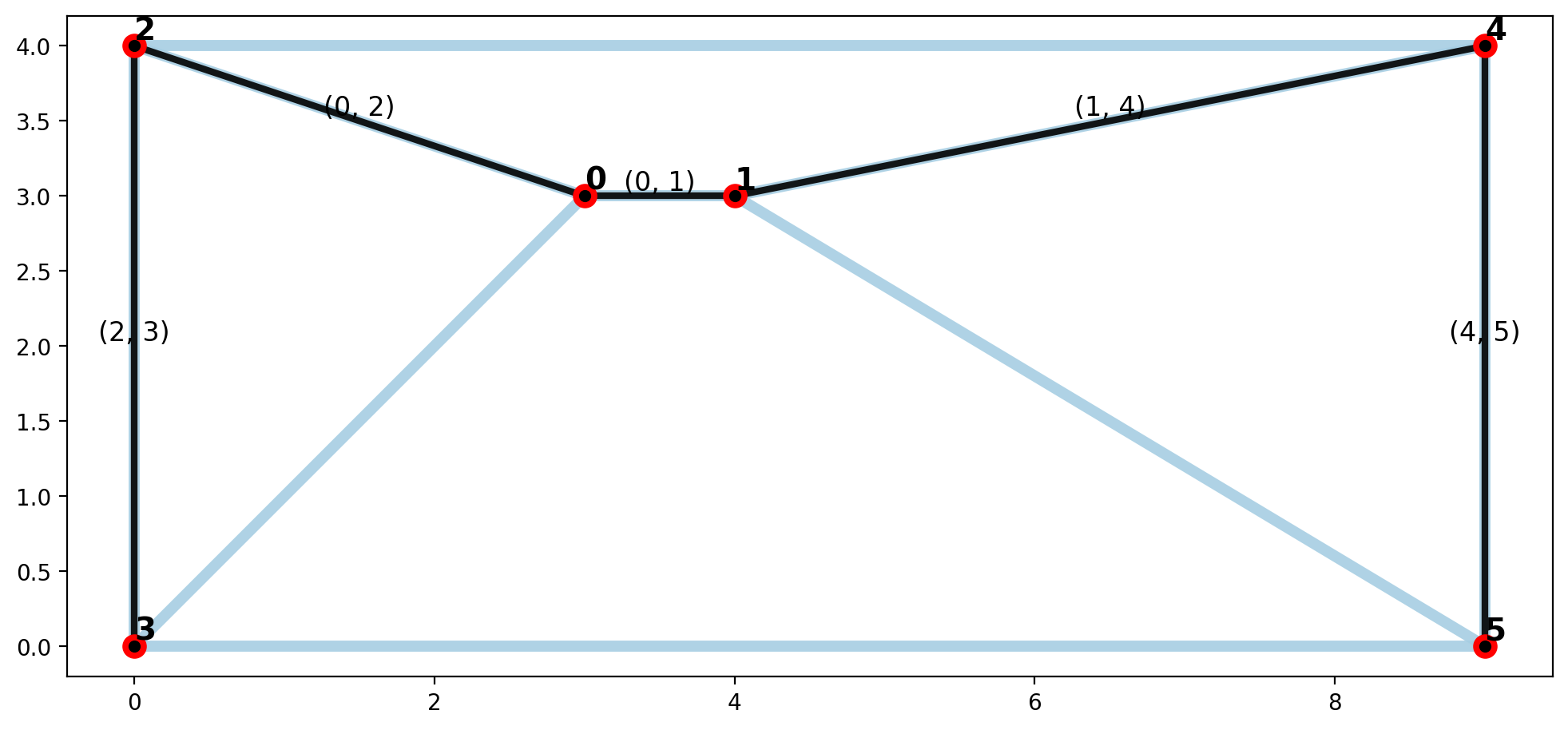Maximum Spanning Tree

:

maxst_net = spaghetti.spanning_tree(ntw, maximum=True)
mst_verts, mst_arcs = spaghetti.element_as_gdf(maxst_net, **elem_kws)
plotter(arcs, vertices, mst_arcs=mst_arcs, mst_verts=mst_verts)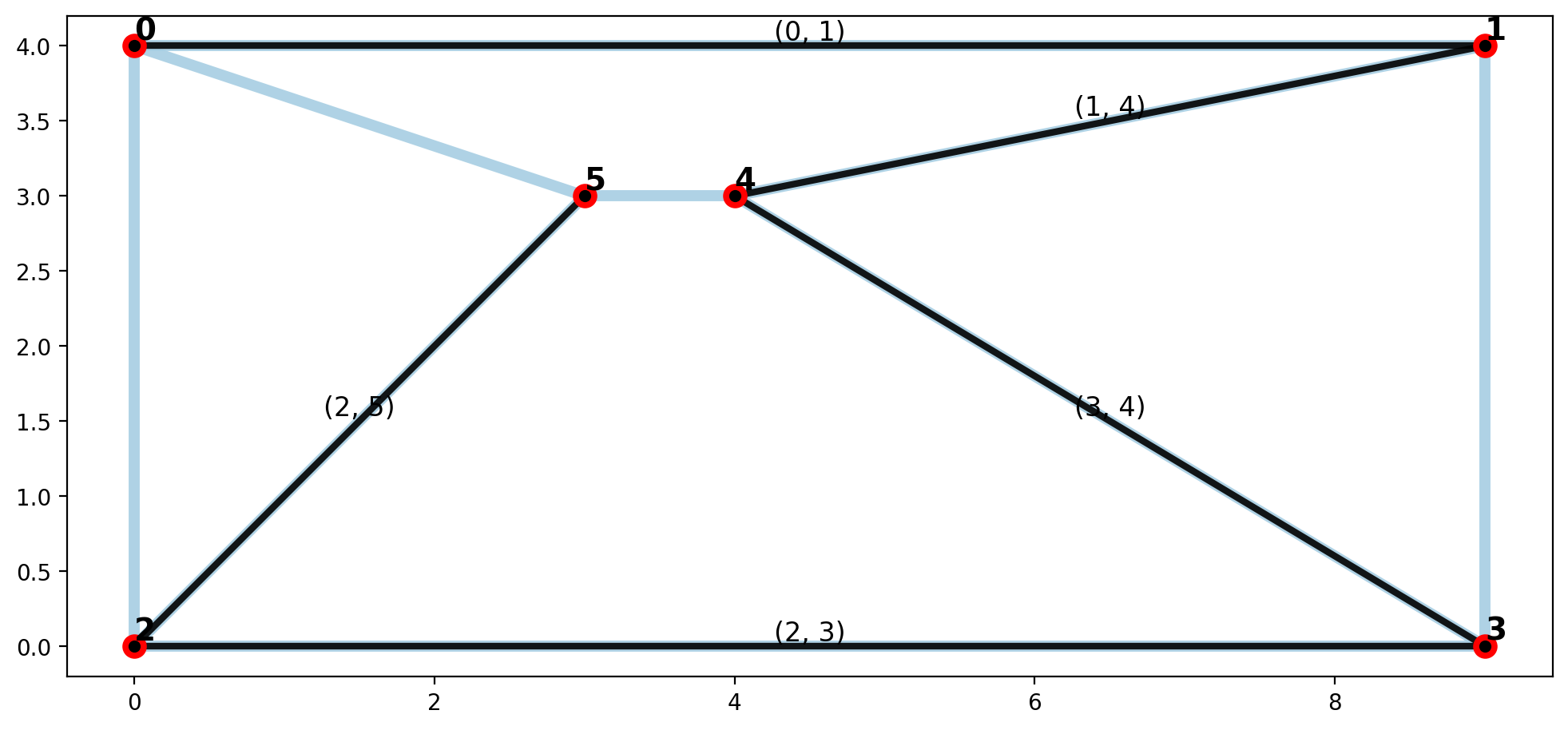### 2.b 4x4 Regular lattice¶

:

bounds = (0,0,3,3)
h, v = 2, 2
lattice = spaghetti.regular_lattice(bounds, h, nv=v, exterior=True)
ntw = spaghetti.Network(in_data=lattice)
vertices, arcs = spaghetti.element_as_gdf(ntw, **elem_kws)

:

plotter(arcs, vertices, mst_arcs=None, mst_verts=None)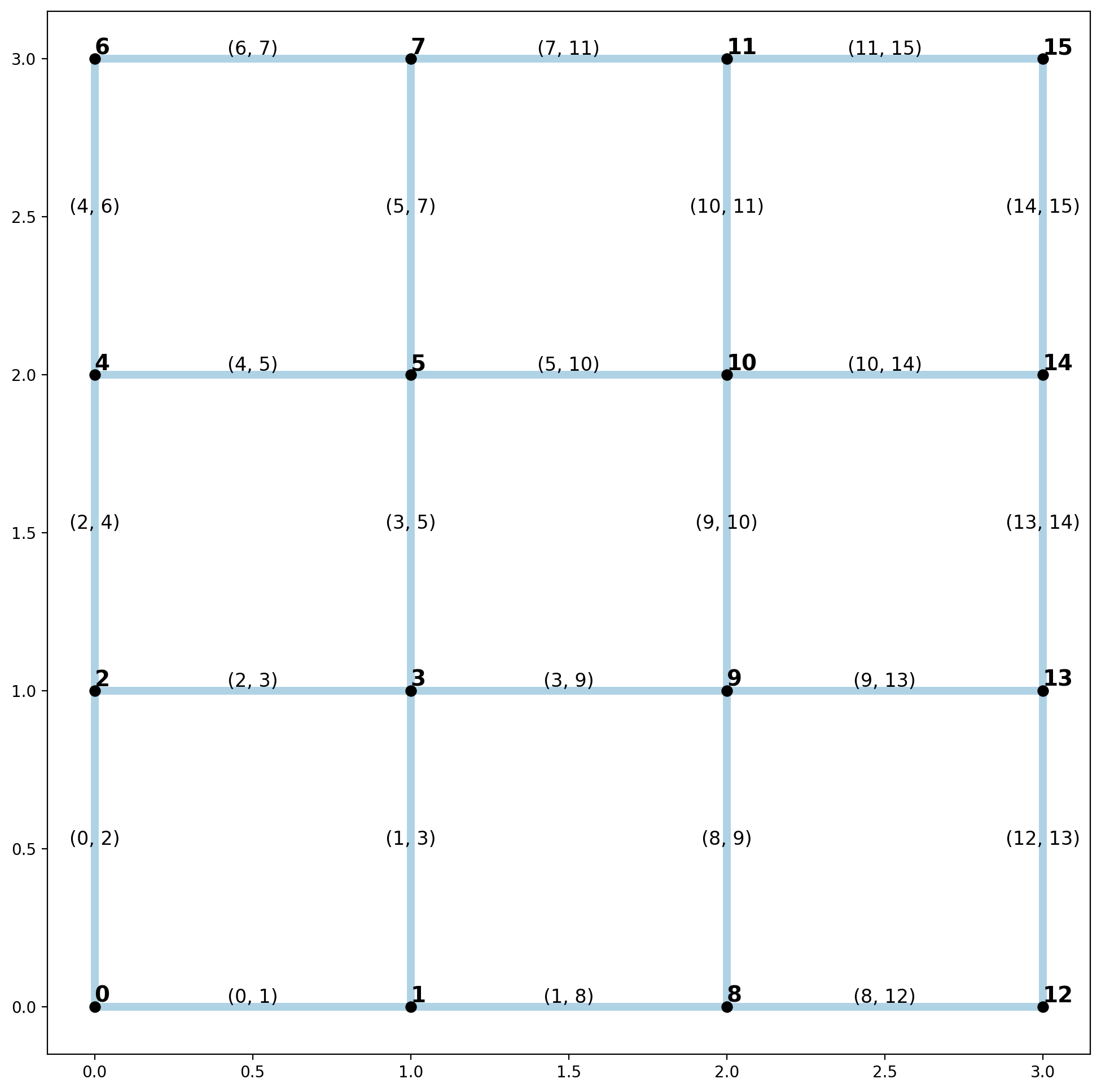Minimum Spanning Tree

:

minst_net = spaghetti.spanning_tree(ntw, maximum=False)
mst_verts, mst_arcs = spaghetti.element_as_gdf(minst_net, **elem_kws)
plotter(arcs, vertices, mst_arcs=mst_arcs, mst_verts=mst_verts)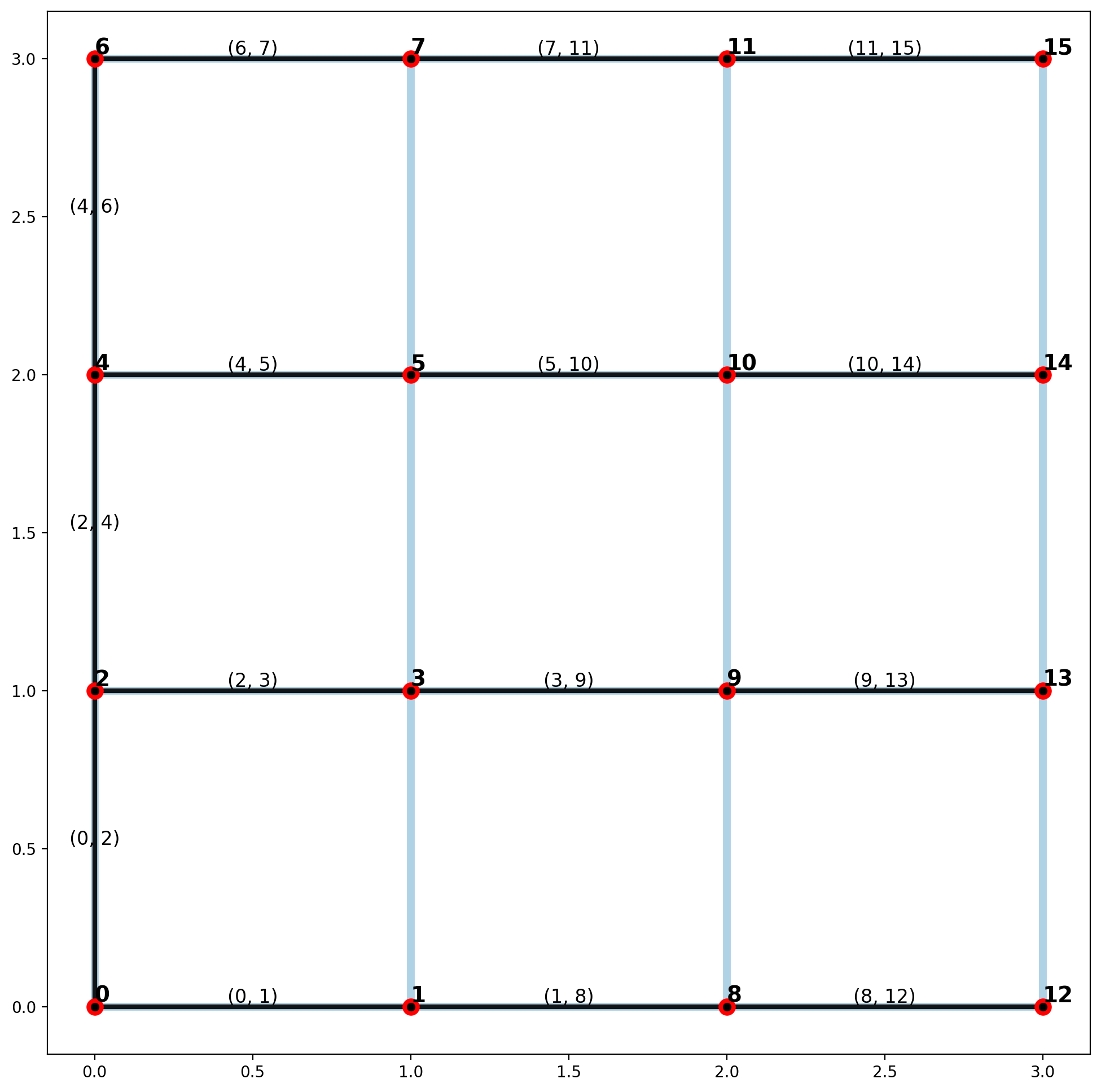Maximum Spanning Tree

:

maxst_net = spaghetti.spanning_tree(ntw, maximum=True)
mst_verts, mst_arcs = spaghetti.element_as_gdf(maxst_net, **elem_kws)
plotter(arcs, vertices, mst_arcs=mst_arcs, mst_verts=mst_verts)Since all network arcs in a regular lattice are equal in length, the minimum and maximum spanning trees will be equivalent, and are dependent on the start index for cycle search.

## 3. Emprical Example — geodanet/streets.shp¶

:

ntw = spaghetti.Network(in_data=libpysal.examples.get_path("streets.shp"))
vertices, arcs = spaghetti.element_as_gdf(ntw, **elem_kws)

:

plotter(arcs, vertices, mst_arcs=None, mst_verts=None, label=False)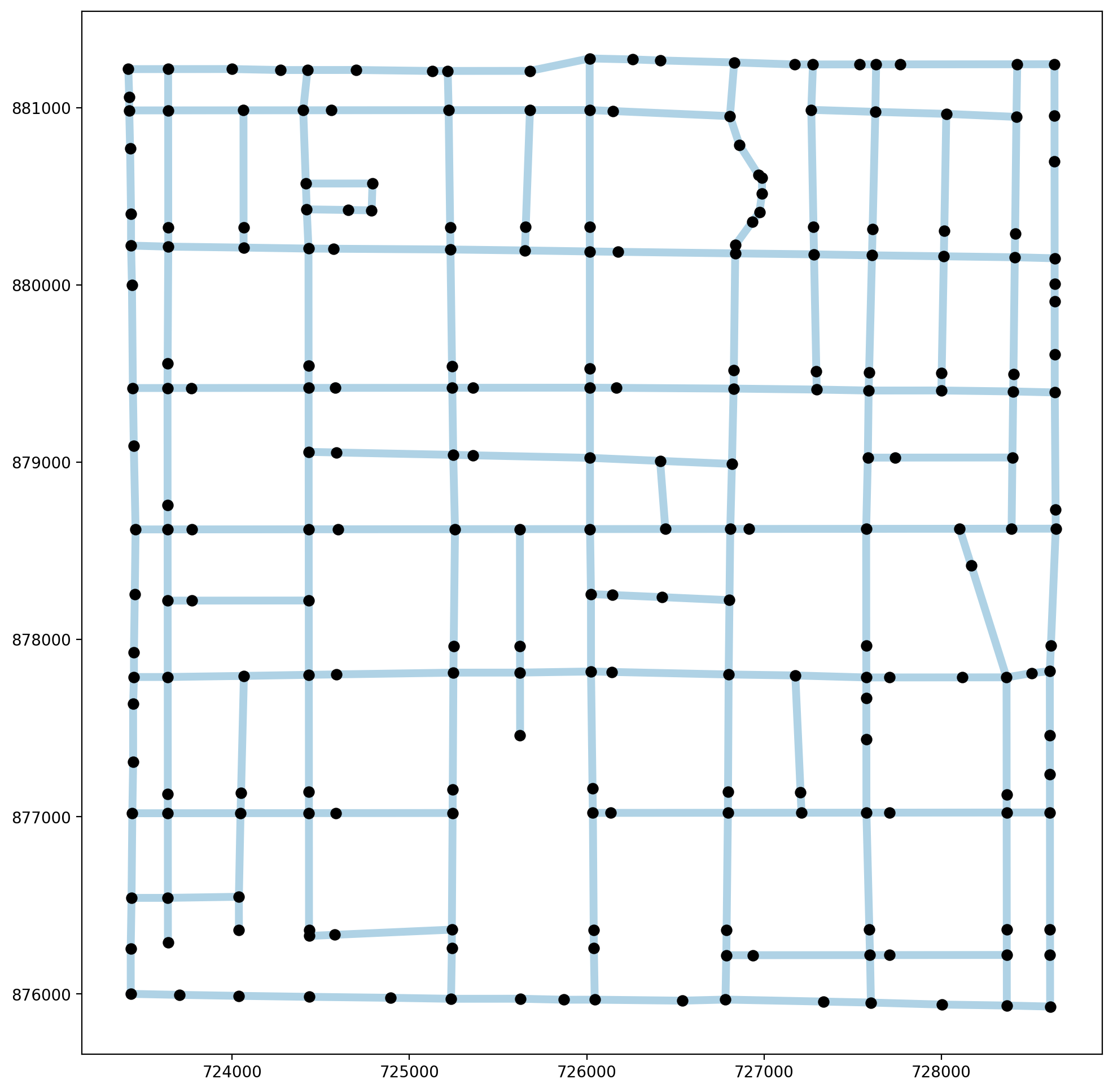Minimum Spanning Tree

:

minst_net = spaghetti.spanning_tree(ntw)
mst_verts, mst_arcs = spaghetti.element_as_gdf(minst_net, **elem_kws)
plotter(arcs, vertices, mst_arcs=mst_arcs, mst_verts=mst_verts, label=False)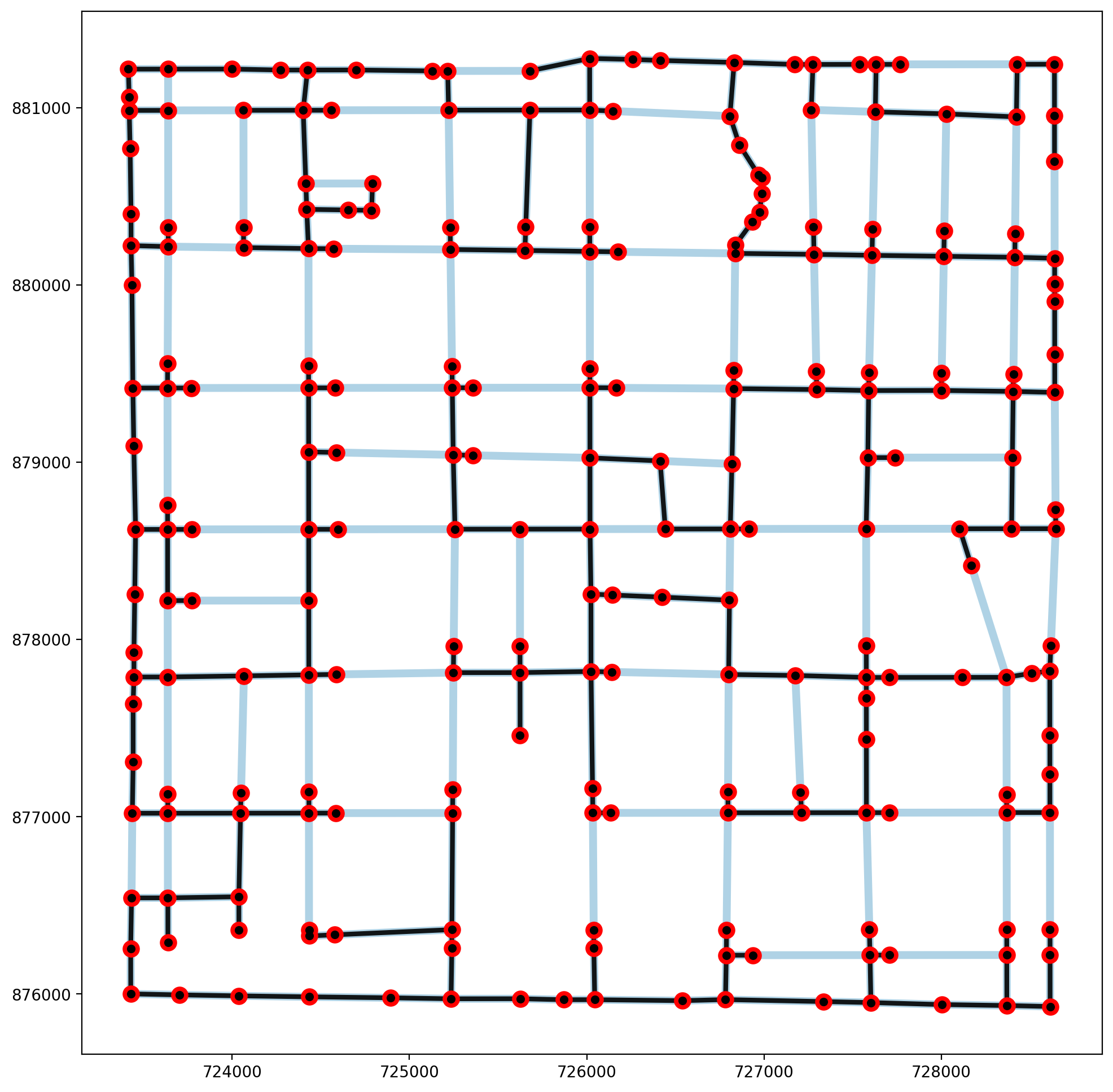Maximum Spanning Tree

:

maxst_net = spaghetti.spanning_tree(ntw, maximum=True)
mst_verts, mst_arcs = spaghetti.element_as_gdf(maxst_net, **elem_kws)
plotter(arcs, vertices, mst_arcs=mst_arcs, mst_verts=mst_verts, label=False)